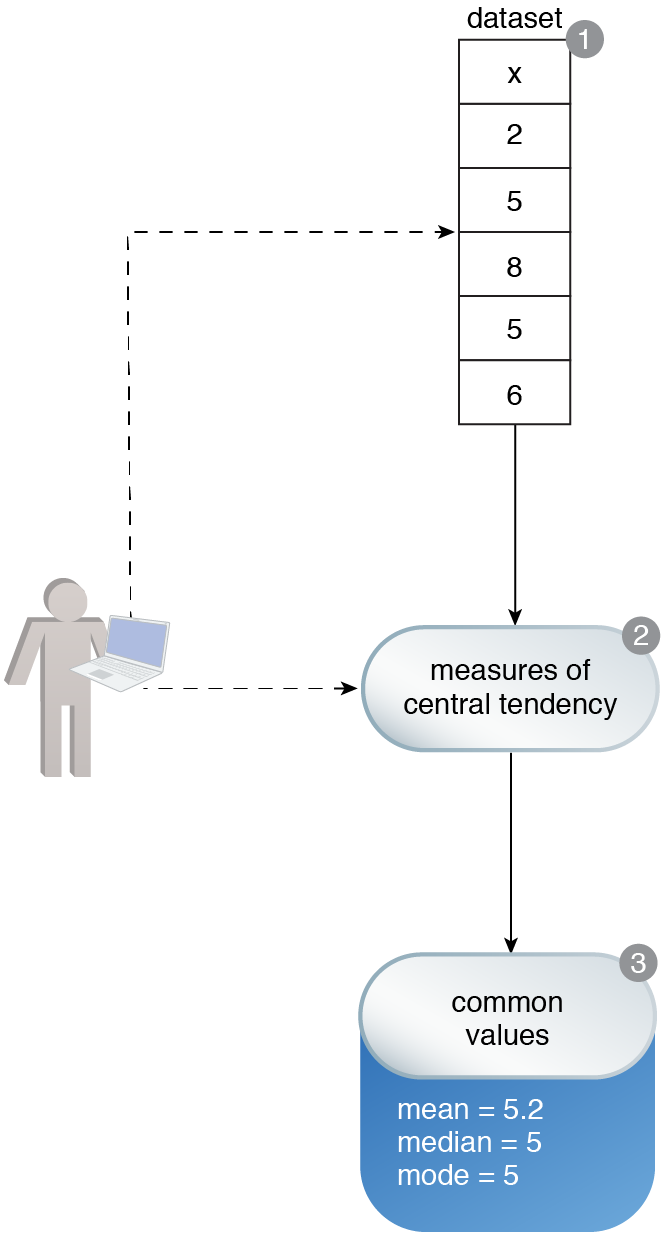# Central Tendency Computation

Machine Learning Patterns, Mechanisms > Data Exploration Patterns > Central Tendency Computation

## Central Tendency Computation (Khattak)

How can the make-up of a dataset be determined in terms of the normal set of values?

### Problem

Before solving a machine learning problem, a preliminary understanding of the input data is required. However, not knowing which techniques to start with can negatively impact the subsequent model development.

### Solution

The dataset is analyzed and the values that normally occur around the center of a distribution as well the most occurring values are calculated via established statistical techniques.

### Application

The dataset is arranged in ascending or descending order, and the measures of central tendency (mean, median, and mode) are calculated.

### Mechanisms

Query Engine, Processing Engine, Resource Manager, Storage DeviceVariable x belongs to a dataset. An understanding of the value of variable x is required (1). To develop this understanding, the measures of central tendency are found (2). Based on the values of the mean, median and mode, it is determined that the most common value is 5 (3).